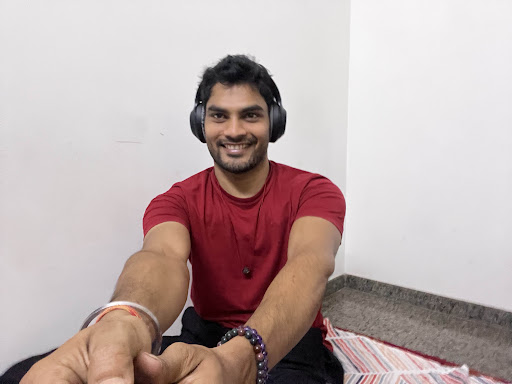# A watch which gains 5 seconds in 3 minutes was set right at 7 a.m. In the afternoon of the same day, when the watch indicated quarter past 4 o'clock, the true time is:

A. $$59\frac{7}{{12}}$$ min. past 3

B. 4 p.m.

C. $$58\frac{7}{{11}}$$ min. past 3

D. $$2\frac{3}{{11}}$$ min. past 4

### Solution(By Examveda Team)

Time from 7 a.m. to 4:15 p.m. = 9 hrs 15 min. = $$\frac{{37}}{4}$$ hrs
3 min. 5 sec. of this c;ocl = 3 min. of the correct clock
⇒ $$\frac{{37}}{{720}}$$ hrs. of this clock = $$\frac{1}{{20}}$$ hrs of the correct clock
⇒ $$\frac{{37}}{4}$$ hrs. of this clock = $$\left( {\frac{1}{{20}} \times \frac{{720}}{{37}} \times \frac{{37}}{4}} \right)$$     hrs. of the correct clock
= 9 hrs. of the correct clock
∴ The correct time is 9 hrs. after 7 a.m. i.e., 4 p.m.

1.2.